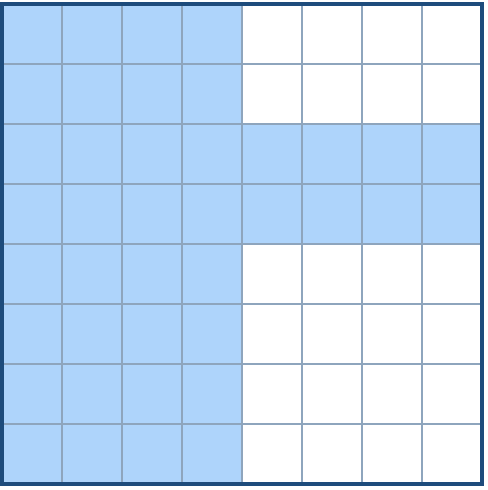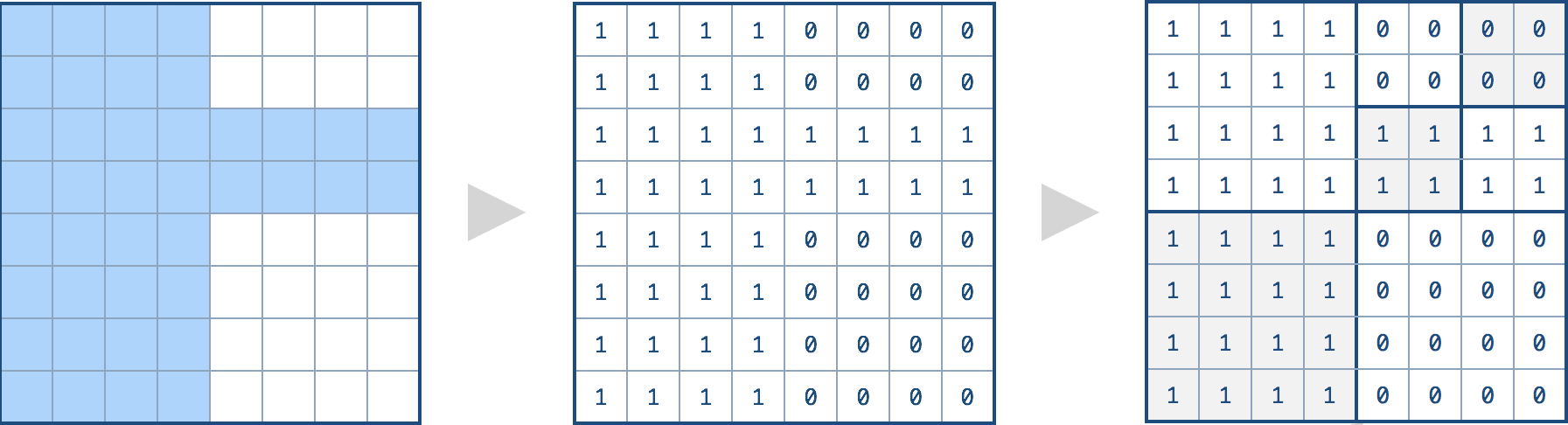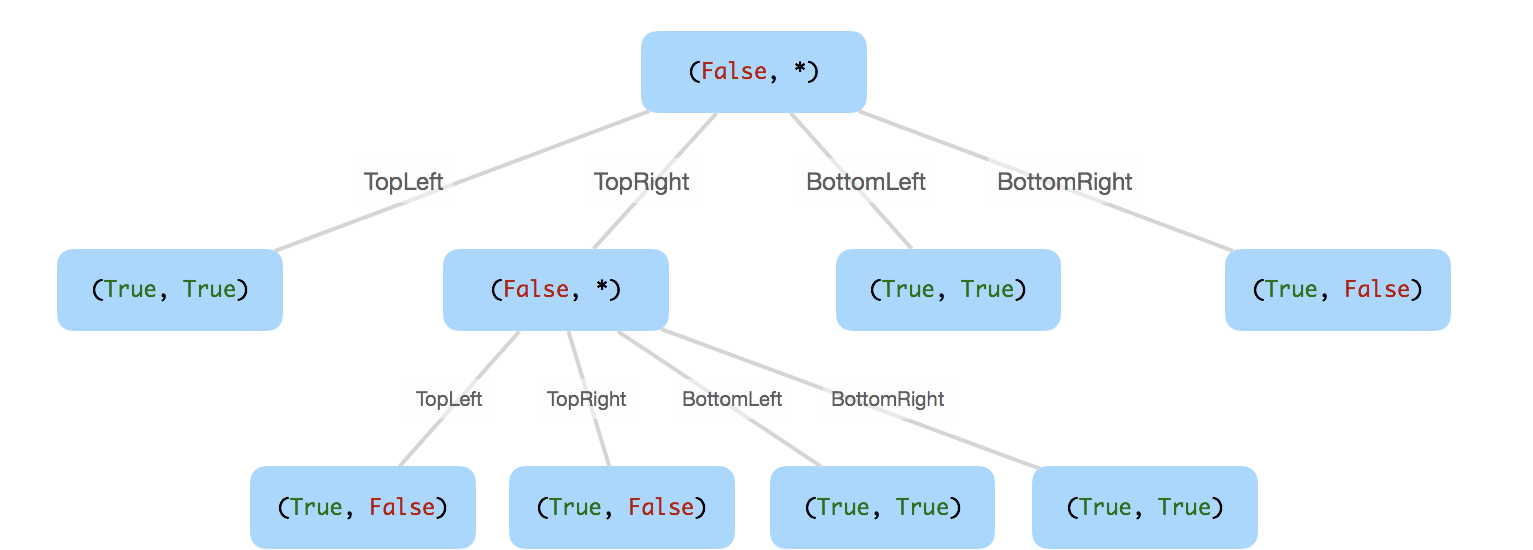#### QUESTION:

We want to use quad trees to store an `N x N` boolean grid. Each cell in the grid can only be true or false. The root node represents the whole grid. For each node, it will be subdivided into four children nodes until the values in the region it represents are all the same.

Each node has another two boolean attributes : `isLeaf` and `val`. `isLeaf` is true if and only if the node is a leaf node. The `val` attribute for a leaf node contains the value of the region it represents.

Given the `8 x 8` grid below, we want to construct the corresponding quad tree:It can be divided according to the definition above:The corresponding quad tree should be as following, where each node is represented as a `(isLeaf, val)` pair.

For the non-leaf nodes, `val` can be arbitrary, so it is represented as `*`.Note:

1. `N` is less than `1000` and guaranteened to be a power of 2.
2. If you want to know more about the quad tree, you can refer to its wiki.

#### EXPLANATION:

1.首先不管怎么样先对整个进行4等分的判断。

2.如果都相同，那么这个节点就是leaf节点，并且值也是可以算出来的。

3.如果不相同，那么就进行递归。

#### SOLUTION:

``````/*
// Definition for a QuadTree node.
class Node {
public boolean val;
public boolean isLeaf;
public Node topLeft;
public Node topRight;
public Node bottomLeft;
public Node bottomRight;

public Node() {}

public Node(boolean _val,boolean _isLeaf,Node _topLeft,Node _topRight,Node _bottomLeft,Node _bottomRight) {
val = _val;
isLeaf = _isLeaf;
topLeft = _topLeft;
topRight = _topRight;
bottomLeft = _bottomLeft;
bottomRight = _bottomRight;
}
};
*/
class Solution {
public Node construct(int[][] grid) {
Node root = new Node();
root.isLeaf = true;
root.val = grid == 1;
constructHelper(grid,0,grid.length,0,grid.length,root);
return root;
}

public static void constructHelper(int[][] grid,int fromx,int tox,int fromy,int toy,Node node){
boolean topLeft = true;
int tmp = grid[fromx][fromy];
w:for(int i = fromx;i<(tox+fromx)/2;i++){
for(int j = fromy;j<(toy+fromy)/2;j++){
if(grid[i][j]!=tmp) {
topLeft = false;
break w;
}
}
}
Node topLeftNode = new Node();
node.topLeft = topLeftNode;
if(!topLeft){
constructHelper(grid,fromx,(tox+fromx)/2,fromy,(toy+fromy)/2,topLeftNode);
}else{
topLeftNode.isLeaf = true;
topLeftNode.val = tmp==1;
}
boolean topRight = true;
tmp = grid[fromx][(toy+fromy)/2];
w: for(int i = fromx;i<(tox+fromx)/2;i++){
for(int j = (toy+fromy)/2;j<toy;j++){
if(grid[i][j]!=tmp){
topRight = false;
break w;
}
}
}
Node topRightNode = new Node();
node.topRight = topRightNode;
if(!topRight){
constructHelper(grid,fromx,(tox+fromx)/2,(toy+fromy)/2,toy,topRightNode);
}else {
topRightNode.isLeaf = true;
topRightNode.val = tmp==1;
}
boolean bottomLeft = true;
tmp = grid[(tox+fromx)/2][fromy];
w: for(int i = (tox+fromx)/2;i<tox;i++){
for(int j = fromy;j<(toy+fromy)/2;j++){
if(grid[i][j]!=tmp){
bottomLeft = false;
break w;
}
}
}
Node bottomLeftNode = new Node();
node.bottomLeft = bottomLeftNode;
if(!bottomLeft){
constructHelper(grid,(tox+fromx)/2,tox,fromy,(toy+fromy)/2,bottomLeftNode);
}else{
bottomLeftNode.isLeaf = true;
bottomLeftNode.val = tmp==1;
}
boolean bottomRight = true;
tmp = grid[(tox+fromx)/2][(toy+fromy)/2];
w: for(int i = (tox+fromx)/2;i<tox;i++){
for(int j = (toy+fromy)/2;j<toy;j++){
if(grid[i][j]!=tmp){
bottomRight = false;
break w;
}
}
}
Node bottomRightNode = new Node();
node.bottomRight = bottomRightNode;
if(!bottomRight){
constructHelper(grid,(tox+fromx)/2,tox,(toy+fromy)/2,toy,bottomRightNode);
}else{
bottomRightNode.isLeaf = true;
bottomRightNode.val = tmp==1;
}

if(!topLeft || !topRight || !bottomLeft||!bottomRight){
node.isLeaf = false;
}
}
}
``````
>